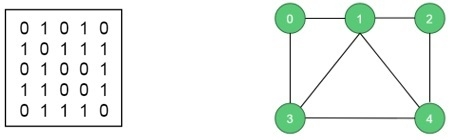# Hamiltonian Cycle

In an undirected graph, the Hamiltonian path is a path, that visits each vertex exactly once, and the Hamiltonian cycle or circuit is a Hamiltonian path, that there is an edge from the last vertex to the first vertex.

In this problem, we will try to determine whether a graph contains a Hamiltonian cycle or not. And when a Hamiltonian cycle is present, also print the cycle.

## Input and Output

Input:
The adjacency matrix of a graph G(V, E).Output:
The algorithm finds the Hamiltonian path of the given graph. For this case it is (0, 1, 2, 4, 3, 0). This graph has some other Hamiltonian paths.
If one graph has no Hamiltonian path, the algorithm should return false.

## Algorithm

isValid(v, k)

Input − Vertex v and position k.

Output − Checks whether placing v in the position k is valid or not.

Begin
if there is no edge between node(k-1) to v, then
return false
if v is already taken, then
return false
return true; //otherwise it is valid
End

cycleFound(node k)

Input − node of the graph.

Output − True when there is a Hamiltonian Cycle, otherwise false.

Begin
if all nodes are included, then
if there is an edge between nodes k and 0, then
return true
else
return false;

for all vertex v except starting point, do
if isValid(v, k), then //when v is a valid edge
if cycleFound(k+1) is true, then
return true
otherwise remove v from the path
done
return false
End

## Example

#include<iostream>
#define NODE 5
using namespace std;

int graph[NODE][NODE] = {
{0, 1, 0, 1, 0},
{1, 0, 1, 1, 1},
{0, 1, 0, 0, 1},
{1, 1, 0, 0, 1},
{0, 1, 1, 1, 0},
};

/* int graph[NODE][NODE] = {
{0, 1, 0, 1, 0},
{1, 0, 1, 1, 1},
{0, 1, 0, 0, 1},
{1, 1, 0, 0, 0},
{0, 1, 1, 0, 0},
}; */

int path[NODE];

void displayCycle() {
cout<<"Cycle: ";

for (int i = 0; i < NODE; i++)
cout << path[i] << " ";
cout << path << endl;      //print the first vertex again
}

bool isValid(int v, int k) {
if (graph [path[k-1]][v] == 0)   //if there is no edge
return false;

for (int i = 0; i < k; i++)   //if vertex is already taken, skip that
if (path[i] == v)
return false;
return true;
}

bool cycleFound(int k) {
if (k == NODE) {             //when all vertices are in the path
if (graph[path[k-1]][ path ] == 1 )
return true;
else
return false;
}

for (int v = 1; v < NODE; v++) {       //for all vertices except starting point
if (isValid(v,k)) {                //if possible to add v in the path
path[k] = v;
if (cycleFound (k+1) == true)
return true;
path[k] = -1;               //when k vertex will not in the solution
}
}
return false;
}

bool hamiltonianCycle() {
for (int i = 0; i < NODE; i++)
path[i] = -1;
path = 0; //first vertex as 0

if ( cycleFound(1) == false ) {
cout << "Solution does not exist"<<endl;
return false;
}

displayCycle();
return true;
}

int main() {
hamiltonianCycle();
}

## Output

Cycle: 0 1 2 4 3 0